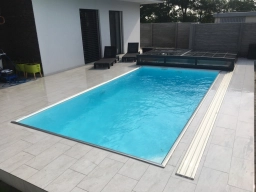# Swimming pool 7

The perimeter of a rectangular swimming pool is 20 4/5 meters. Its length is thrice its width. What is the length of the pool? What is its width?

a =  7 45 = 39/5 m
b =  2 35 = 13/5 m

### Step-by-step explanation:Did you find an error or inaccuracy? Feel free to write us. Thank you!

Tips for related online calculators
Need help with mixed numbers? Try our mixed-number calculator.
Do you have a linear equation or system of equations and looking for its solution? Or do you have a quadratic equation?

#### Grade of the word problem:

We encourage you to watch this tutorial video on this math problem: